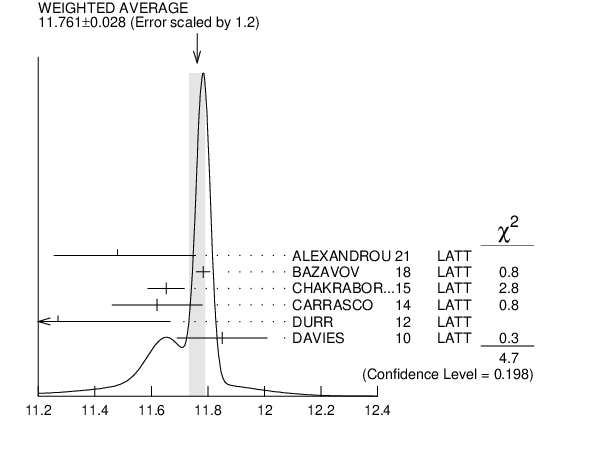#### ${\boldsymbol m}_{{{\boldsymbol c}}}/{\boldsymbol m}_{{{\boldsymbol s}}}$ MASS RATIO

VALUE DOCUMENT ID TECN
 $\bf{ 11.72 \pm0.25}$ OUR EVALUATION See the ideogram below.
$11.783$ $\pm0.025$ 1
 2018
LATT
$11.652$ $\pm0.065$ 2
 2015
LATT
$11.62$ $\pm0.16$ 3
 2014
LATT
$11.27$ $\pm0.30$ $\pm0.26$ 4
 2012
LATT
$11.85$ $\pm0.16$ 5
 2010
LATT
• • We do not use the following data for averages, fits, limits, etc. • •
$11.747$ $\pm0.019$ ${}^{+0.059}_{-0.043}$ 6
 2014 A
LATT
$12.0$ $\pm0.3$ 7
 2010
LATT
 1 BAZAVOV 2018 determine the quark masses using a lattice computation with staggered fermions and four active quark flavors.
 2 CHAKRABORTY 2015 is a lattice QCD computation on gluon field configurations with 2+1+1 dynamical flavors of HISQ quarks with u/d masses down to the physical value. ${\mathit m}_{{{\mathit c}}}$ and ${\mathit m}_{{{\mathit s}}}$ are tuned from pseudoscalar meson masses.
 3 CARRASCO 2014 is a lattice QCD computation of light quark masses using 2 + 1 + 1 dynamical quarks, with ${{\mathit m}_{{u}}}$ = ${{\mathit m}_{{d}}}{}\not=$ ${{\mathit m}_{{s}}}{}\not=$ ${{\mathit m}_{{c}}}$. The ${\mathit {\mathit u}}$ and ${\mathit {\mathit d}}$ quark masses are obtained separately by using the ${{\mathit K}}$ meson mass splittings and lattice results for the electromagnetic contributions.
 4 DURR 2012 determine ${\mathit m}_{{{\mathit c}}}/{\mathit m}_{{{\mathit s}}}$ using a lattice computation with ${{\mathit N}_{{f}}}$ = 2 dynamical fermions. The result is combined with other determinations of ${\mathit m}_{{{\mathit c}}}$ to obtain ${\mathit m}_{{{\mathit s}}}$(2 GeV) = $97.0$ $\pm2.6$ $\pm2.5~$MeV.
 5 DAVIES 2010 determine ${\mathit m}_{{{\mathit c}}}/{\mathit m}_{{{\mathit s}}}$ from meson masses calculated on gluon fields including ${{\mathit u}}$, ${{\mathit d}}$, and ${{\mathit s}}$ sea quarks with lattice spacing down to 0.045 fm. The Highly Improved Staggered quark formalism is used for the valence quarks.
 6 BAZAVOV 2014A is a lattice computation using 4 dynamical flavors of HISQ fermions.
 7 BLOSSIER 2010 determine ${\mathit m}_{{{\mathit c}}}/{\mathit m}_{{{\mathit s}}}$ from a computation of the hadron spectrum using ${{\mathit N}_{{f}}}$ = 2 dynamical twisted-mass Wilson fermions.${\mathit m}_{{{\mathit c}}}/{\mathit m}_{{{\mathit s}}}$ MASS RATIO
References:
 BAZAVOV 2018
PR D98 054517 Up-, down-, strange-, charm-, and bottom-quark masses from four-flavor lattice QCD
 CHAKRABORTY 2015
PR D91 054508 High-Precision Quark Masses and QCD Coupling from ${{\mathit n}_{{f}}}$ = 4 Lattice QCD
 BAZAVOV 2014A
PR D90 074509 Charmed and Light Pseudoscalar Meson Decay Constants from Four-flavor Lattice QCD with Physical Light Quarks
 CARRASCO 2014
NP B887 19 Up, Down, Strange and Charm Quark Masses with $\mathit N_{f}$ = 2+1+1 Twisted Mass Lattice QCD
 DURR 2012
PRL 108 122003 Ratio ${\mathit m}_{{{\mathit c}}}/{\mathit m}_{{{\mathit s}}}$ with Wilson Fermions
 BLOSSIER 2010
PR D82 114513 Average up/down, strange, and charm Quark Masses with $\mathit N_{f}$=2 Twisted-Mass Lattice QCD
 DAVIES 2010
PRL 104 132003 Precise Charm to Strange Mass Ratio and Light Quark Masses from Full Lattice QCD